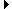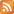Rietveld Code Review Tool
Help | Bug tracker | Discussion group | Source code | Sign in
(618)

## Issue 1179044: Python: Equality and hashing for functools.partial

Can't Edit
Can't Publish+Mail
Start Review
Created:
13 years, 4 months ago by DUrban
Modified:
10 months, 1 week ago
Reviewers:
Éz
Base URL:
http://svn.python.org/view/*checkout*/python/branches/py3k/
Visibility:
Public.

### Description

Python feature request: http://bugs.python.org/issue8699 On python-dev came up an idea  to support equality (== and !=) and hashing by functools.partial instances. Van Lindberg provided an implementation written in Python . I've made a very similar implementation in C (in Modules/_functoolsmodule.c). The Python equivalent of my code is in Lib/test/test_functools.py as the PythonPartialCls class. The hashing differs a little from Van Lindberg's implementation: I'm computing the "normal form" of the dict as the sorted list of its items (not as the sorted list of the keys followed by the items). (It was easier to implement this way.) I haven't made a lot of Python programming in C, so I'm not sure I made everything in the right way (especially the reference counting). I'd appreciate every suggestion, and will try to correct my mistakes. Thanks!  http://mail.python.org/pipermail/python-dev/2010-May/099981.html  http://mail.python.org/pipermail/python-dev/2010-May/099996.html

### Patch Set 4 : Corrected version, __eq__ now compares the __dict__ of the two instances. #

Unified diffs Side-by-side diffs Delta from patch set Stats (+198 lines, -4 lines) PatchLib/test/test_functools.py View 1 3 3 chunks +79 lines, -0 lines 2 comments DownloadModules/_functoolsmodule.c View 1 3 6 chunks +119 lines, -4 lines 1 comment Download

### Messages

Total messages: 5
DUrban 13 years, 4 months ago (2010-05-12 15:05:17 UTC) #1
DUrban
 Here is a corrected version (using PyList_Sort to sort the list).
13 years, 4 months ago (2010-05-13 07:27:42 UTC) #2
DUrban
 Corrected version, with read-only keywords dictionary.
13 years, 4 months ago (2010-05-13 16:41:34 UTC) #3
DUrban
 Corrected version, __eq__ now compares the __dict__ of the two instances.
13 years, 4 months ago (2010-05-16 12:22:30 UTC) #4
Éz
 Index: Lib/test/test_functools.py =================================================================== --- Lib/test/test_functools.py (revision 81228) +++ Lib/test/test_functools.py (working copy) @@ -17,6 +17,39 @@ ...
10 months, 1 week ago (2022-11-18 14:37:05 UTC) #5
```Index: Lib/test/test_functools.py
===================================================================
--- Lib/test/test_functools.py	(revision 81228)
+++ Lib/test/test_functools.py	(working copy)
@@ -17,6 +17,39 @@
newfunc.keywords = keywords
return newfunc

+class PythonPartialCls:
+
+    """Pure Python approximation of partial()"""
+
+    def __init__(self, func, *args, **keywords):
+        self.func = func
+        self.args = args
+        self.keywords = keywords
+
+    def __call__(self, *fargs, **fkeywords):
+        newkeywords = self.keywords.copy()
+        newkeywords.update(fkeywords)
+        return self.func(*(self.args + fargs), **newkeywords)
+
+    def __eq__(self, other):
+        if isinstance(other, PythonPartialCls):
+            return ((self.func is other.func) and
+                    (self.args == other.args) and
+                    (self.keywords == other.keywords) and
+                    (self.__dict__ == other.__dict__))
+        else:
+            return NotImplemented
+
+    def __hash__(self):
+        if self.keywords is None:
+            kwhash = None
+        else:
+            itemlist = list(self.keywords.items())
+            itemlist.sort()
+            kwhash = tuple(itemlist)
+        return hash((self.func, self.args, kwhash))
+
+
def capture(*args, **kw):
"""capture all positional and keyword arguments"""
return args, kw
@@ -151,6 +184,43 @@
self.assertEqual(signature(f), signature(f_copy))

+    def test_equality(self):
+        f1 = self.thetype(capture, 1, {'a', 'b'}, a=2, b={'a': 1, 'b': 2})
+        f2 = self.thetype(capture, 1, {'b', 'a'}, a=2, b={'b': 2, 'a': 1})
+        self.assert_(f1 == f2)
+        self.assert_(not (f1 != f2))
+        # __dict__ different:
+        f2.foo = 'bar'
+        self.assert_(f1 != f2)
+        self.assert_(not (f1 == f2))
+        f1.foo = 'baz'
+        self.assert_(f1 != f2)
+        self.assert_(not (f1 == f2))
+        # func different:
+        f1 = self.thetype(capture, 1, {'a', 'b'}, a=2, b={'a': 1, 'b': 2})
+        f2 = self.thetype(signature, 1, {'b', 'a'}, a=2, b={'b': 2, 'a': 1})
+        self.assert_(f1 != f2)
+        self.assert_(not (f1 == f2))
+        # args different:
+        f2 = self.thetype(capture, 1, {'b', 'x'}, a=2, b={'b': 2, 'a': 1})
+        self.assert_(f1 != f2)
+        self.assert_(not (f1 == f2))
+        # kwargs different:
+        f2 = self.thetype(capture, 1, {'b', 'a'}, a=2, b={'b': 2, 'a': 9})
+        self.assert_(f1 != f2)
+        self.assert_(not (f1 == f2))
+        # == with some other object:
+        self.assert_(f1 != object())
+        self.assert_(not (f1 == object()))
+
+    def test_hash(self):
+        f1 = self.thetype(capture, 1, a=2, b=3)
+        f2 = self.thetype(capture, 1, b=3, a=2)
+        self.assertEqual(hash(f1), hash(f2))
+        # immutable hash value:
+        f2.foo = 'bar'
+        self.assertEqual(hash(f1), hash(f2))
+
class PartialSubclass(functools.partial):
pass

@@ -165,6 +235,15 @@
# the python version isn't picklable
def test_pickle(self): pass

+    # for eq and hash test we need to use the class implementation:
+    def test_equality(self):
+        self.thetype = PythonPartialCls
+        super().test_equality()
+
+    def test_hash(self):
+        self.thetype = PythonPartialCls
+        super().test_equality()
+
class TestUpdateWrapper(unittest.TestCase):

def check_wrapper(self, wrapper, wrapped,
Index: Modules/_functoolsmodule.c
===================================================================
--- Modules/_functoolsmodule.c	(revision 81228)
+++ Modules/_functoolsmodule.c	(working copy)
@@ -84,6 +84,50 @@
Py_TYPE(pto)->tp_free(pto);
}

+static long
+partial_hash(partialobject *pto)
+{
+    PyObject *kwhash;
+    PyObject *itemlist;
+    PyObject *tuple;
+    long hash;
+
+    if (pto->kw == Py_None) {
+        kwhash = Py_None;
+        Py_INCREF(Py_None);
+    }
+    /* pto->kw is a dict, but it isn't really mutable,
+       so we convert it to a sorted list of its items. */
+    else {
+        /* Get the items as a list: */
+        itemlist = PySequence_List(PyDict_Items(pto->kw));
+        if(itemlist == NULL)
+            return -1;
+
+        /* Sort it: */
+        if (PyList_Sort(itemlist) == -1) {
+            Py_DECREF(itemlist);
+            return -1;
+        }
+
+        /* Convert the sorted list to a tuple (because that is hashable): */
+        kwhash = PyList_AsTuple(itemlist);
+        Py_DECREF(itemlist);
+        if (kwhash == NULL)
+            return -1;
+    }
+
+    /* Return the hash of a tuple: (func, args, kwhash) */
+    tuple = Py_BuildValue("(OOO)", pto->fn, pto->args, kwhash);
+    Py_DECREF(kwhash);
+    if (tuple == NULL) {
+        return -1;
+    }
+    hash = PyObject_Hash(tuple);
+    Py_DECREF(tuple);
+    return hash;
+}
+
static PyObject *
partial_call(partialobject *pto, PyObject *args, PyObject *kw)
{
@@ -140,6 +184,63 @@
return 0;
}

+static PyObject *
+partial_richcompare(PyObject *left, PyObject *right, int op)
+{
+    int result;
+    partialobject *l_pto;
+    partialobject *r_pto;
+    PyObject *l_dict;
+    PyObject *r_dict;
+
+    if (PyType_IsSubtype(Py_TYPE(right), &partial_type) && (op == Py_EQ || op
== Py_NE)) {
+        l_pto = (partialobject *)left;
+        r_pto = (partialobject *)right;
+        if (l_pto->fn == r_pto->fn) {
+            /* Compare args: */
+            result = PyObject_RichCompareBool(l_pto->args, r_pto->args, Py_EQ);
+            if (result == -1)
+                return NULL;
+            /* Compare keywords: */
+            if (result == 1)
+                result = PyObject_RichCompareBool(l_pto->kw, r_pto->kw, Py_EQ);
+            if (result == -1)
+                return NULL;
+            /* Compare __dict__: */
+            if (result == 1 && (l_pto->dict != r_pto->dict)) {
+                l_dict = PyObject_GetAttrString(left, "__dict__");
+                if (l_dict == NULL)
+                    return NULL;
+                r_dict = PyObject_GetAttrString(right, "__dict__");
+                if (r_dict == NULL) {
+                    Py_DECREF(l_dict);
+                    return NULL;
+                }
+                result = PyObject_RichCompareBool(l_dict, r_dict, Py_EQ);
+                Py_DECREF(l_dict);
+                Py_DECREF(r_dict);
+            }
+        }
+        else
+            result = 0;
+
+        if (op == Py_NE)
+            result = (result == 0);
+
+        if(result) {
+            Py_INCREF(Py_True);
+            return Py_True;
+        }
+        else {
+            Py_INCREF(Py_False);
+            return Py_False;
+        }
+    }
+
+    Py_INCREF(Py_NotImplemented);
+    return Py_NotImplemented;
+}
+
PyDoc_STRVAR(partial_doc,
"partial(func, *args, **keywords) - new function with partial application\n\
of the given arguments and keywords.\n");
@@ -150,8 +251,6 @@
"function object to use in future partial calls"},
"tuple of arguments to future partial calls"},
-     "dictionary of keyword arguments to future partial calls"},
{NULL}  /* Sentinel */
};

@@ -191,8 +290,24 @@
return 0;
}

+static PyObject *
+partial_get_keywords(partialobject *pto)
+{
+    PyObject *keywords;
+    if (pto->kw != Py_None) {
+        keywords = PyDictProxy_New(pto->kw);
+    }
+    else {
+        keywords = Py_None;
+        Py_INCREF(Py_None);
+    }
+    return keywords;
+}
+
static PyGetSetDef partial_getsetlist[] = {
{"__dict__", (getter)partial_get_dict, (setter)partial_set_dict},
+    {"keywords", (getter)partial_get_keywords, NULL,
+     "dictionary of keyword arguments to future partial calls"},
{NULL} /* Sentinel */
};

@@ -258,7 +373,7 @@
0,                                  /* tp_as_number */
0,                                  /* tp_as_sequence */
0,                                  /* tp_as_mapping */
-    0,                                  /* tp_hash */
+    (hashfunc)partial_hash,             /* tp_hash */
(ternaryfunc)partial_call,          /* tp_call */
0,                                  /* tp_str */
PyObject_GenericGetAttr,            /* tp_getattro */
@@ -269,7 +384,7 @@
partial_doc,                        /* tp_doc */
(traverseproc)partial_traverse,     /* tp_traverse */
0,                                  /* tp_clear */
-    0,                                  /* tp_richcompare */
+    partial_richcompare,                /* tp_richcompare */
offsetof(partialobject, weakreflist),       /* tp_weaklistoffset */
0,                                  /* tp_iter */
0,                                  /* tp_iternext */

https://codereview.appspot.com/1179044/diff/16001/Lib/test/test_functools.py
File Lib/test/test_functools.py (right):

https://codereview.appspot.com/1179044/diff/16001/Lib/test/test_functools.py#...
Lib/test/test_functools.py:47: itemlist = list(self.keywords.items())
+    """Pure Python approximation of partial()"""
+
+    def __init__(self, func, *args, **keywords):
+        self.func = func
+        self.args = args
+        self.keywords = keywords
+
+    def __call__(self, *fargs, **fkeywords):
+        newkeywords = self.keywords.copy()
+        newkeywords.update(fkeywords)

https://codereview.appspot.com/1179044/diff/16001/Lib/test/test_functools.py#...
Lib/test/test_functools.py:188: f1 = self.thetype(capture, 1, {'a', 'b'}, a=2,
b={'a': 1, 'b': 2})
Test

https://codereview.appspot.com/1179044/diff/16001/Modules/_functoolsmodule.c
File Modules/_functoolsmodule.c (right):

https://codereview.appspot.com/1179044/diff/16001/Modules/_functoolsmodule.c#...
Modules/_functoolsmodule.c:309: {"keywords", (getter)partial_get_keywords, NULL,
Null*kwash<(ítemlíst) rémóve data)```Recent Issues | This issue